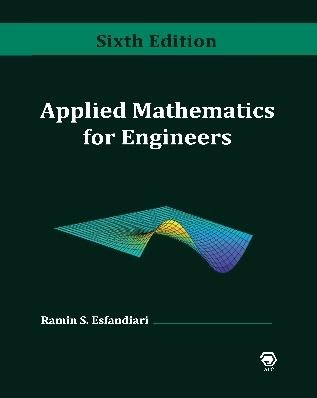## Inside: Applied Mathematics for Engineers, 6th EditionApplied Mathematics for Engineers, 6th Edition

# Preface

The most essential goal of this book is to provide the reader with a comprehensive knowledge of fundamental concepts and methods of applied mathematics utilized in various engineering disciplines. Each topic is covered in great detail, followed by meticulously worked-out examples, as well as a problem set containing a large number of additional related exercises. The book emphasizes on engineering applications to familiarize the reader with the basic physical laws and proper engineering terminology.

The book is divided into three main parts: The first part covers complex analysis (Chapter 1), ordinary and partial differential equations (Chapters 2–4 and 7), Laplace transformation (Chapter 5), and Fourier analysis (Chapter 6).

The second part presents a detailed coverage of matrix analysis (Chapter 8) and matrix eigenvalue problem (Chapter 9).  Several applications in engineering vibrations and modal analysis are included for a better understanding of the effectiveness of matrix computations in determining systems’ natural frequencies and mode shapes.

The third part is completely devoted to numerical methods with applications in various engineering disciplines.  The powerful software MATLAB® is introduced in Chapter 10 and is used to perform symbolic, graphical, and numerical tasks.  Chapter 11 introduces techniques for solving equations and systems of equations.  Chapter 12 discusses curve fitting and interpolation techniques, while Chapter 13 covers numerical differentiation and integration methods.  Chapter 14 presents numerical methods for solving initial- and boundary-value problems.  Chapter 15 introduces methods to solve the matrix eigenvalues problem.  Numerical solution of partial differential equations is covered in Chapter 16.

An ample part of the material in this book has been rigorously class tested over the past several years, and the valuable observations and suggestions made by students and professors who used the earlier editions have greatly contributed to making this new edition as complete and user-friendly as possible.

The 5th edition of the book (2013) has been entirely revamped to produce this latest edition through

• Inclusion of several topics and exclusion of a few others
• Additional details on the presentation of material
• Increased display of hand calculations in the worked-out examples
• More thought-out sets of exercises at the end of each chapter
• Updating the MATLAB codes and functions based on a more recent release (2019b)

Pedagogy of the Book

The book is very user-friendly, in an effort to make the material easy to understand by the reader.  In Chapters 1 through 9, each topic is accompanied by at least one fully worked-out example and a large number of exercises in the problem sets.  In Chapters 10 through 16, the following format is utilized:

• Each method is accompanied by a MATLAB user-defined function or script file to perform a specific task
• This is followed by at least one thoroughly worked-out example showing all details of hand calculations
• The results are then confirmed through the execution of the user-defined function or the script file
• Whenever possible, MATLAB built-in functions are executed for re-confirmation of the results
• Plots are regularly generated to shed light on the accuracy and significance of the numerical results

# Exercises (Chapters 10–16)

A large set of problems/projects with various levels of difficulty is included at the end of each chapter, which can be worked out using either

• hand calculation, or
• MATLAB®

In many instances, the reader is asked to prepare a user-defined function or a script file that implements a specific technique, which often require revisions to those already presented in the chapter.

Ramin S. Esfandiari, Ph.D.

May 2021